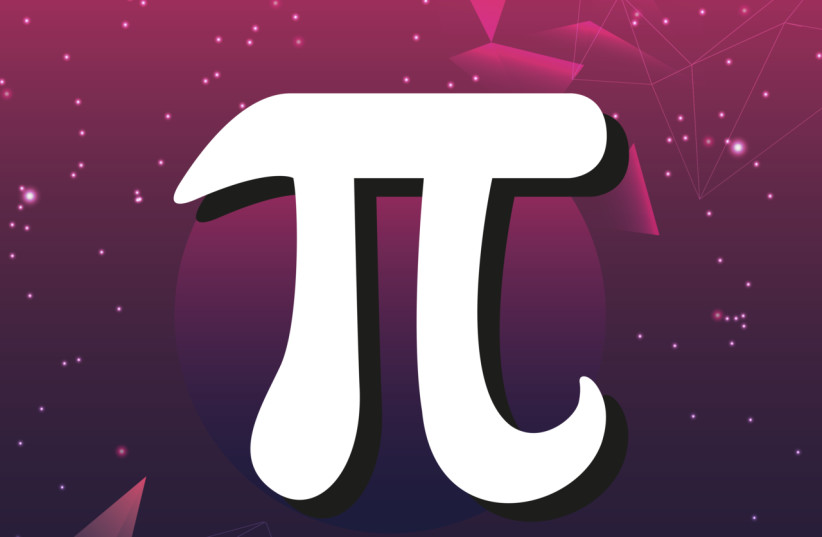(function (a, d, o, r, i, c, u, p, w, m) { m = d.getElementsByTagName(o), a[c] = a[c] || {}, a[c].trigger = a[c].trigger || function () { (a[c].trigger.arg = a[c].trigger.arg || []).push(arguments)}, a[c].on = a[c].on || function () {(a[c].on.arg = a[c].on.arg || []).push(arguments)}, a[c].off = a[c].off || function () {(a[c].off.arg = a[c].off.arg || []).push(arguments) }, w = d.createElement(o), w.id = i, w.src = r, w.async = 1, w.setAttribute(p, u), m.parentNode.insertBefore(w, m), w = null} )(window, document, "script", "https://95662602.adoric-om.com/adoric.js", "Adoric_Script", "adoric","9cc40a7455aa779b8031bd738f77ccf1", "data-key");
var domain=window.location.hostname; var params_totm = ""; (new URLSearchParams(window.location.search)).forEach(function(value, key) {if (key.startsWith('totm')) { params_totm = params_totm +"&"+key.replace('totm','')+"="+value}}); var rand=Math.floor(10*Math.random()); var script=document.createElement("script"); script.src=`https://stag-core.tfla.xyz/pre_onetag?pub_id=34&domain=\${domain}&rand=\${rand}&min_ugl=0\${params_totm}`; document.head.append(script);

# Happy Pi Day: Can you solve NASA's space challenges?

## Pi is essential for studying the universe, so NASA uses it often. Students can solve some real problems related to Pi that NASA had to deal with in the past.In honor of the mathematical constant pi – and its many uses in space exploration – the annual NASA Pi Day Challenge offers four math problems involving real NASA missions and science.
(photo credit: NASA/JPL-Caltech)

In honor of Pi Day, NASA's Jet Propulsion Laboratory (JPL) announced a new series of math challenges involving real space missions that all used the number pi.

Pi Day, which falls on March 14 every year due to the date being abbreviated as 3.14, is a day that commemorates the famous and perplexing mathematical constant of pi.

What is pi? Aside from referring to a rather enjoyable desert, pi is a very important number in mathematics, an infinite series of decimals that is usually rounded to the first three numbers: 3.14.

This digit is used in a wide array of different mathematical applications, such as finding the circumference of a circle.

And what's more: NASA uses it a lot, whether for missions to study Earth or Mars or asteroids. Pi is essential for studying the universe.Pi (illustrative). (credit: Wikimedia Commons)

This is why NASA has made an annual Pi Day Challenge in celebration of pi, where, for the 10th year, students everywhere can use math to solve some real problems NASA has faced in the past involving pi.

Here are the questions NASA is asking this year.

### What are the four challenge questions for NASA's Pi Day?

1. Calculate the volume of a Martian rock core gathered by NASA's Perseverance rover
2. Understand the cosmos-peering power of the Hubble Space Telescope and James Webb Space Telescope by comparing the  area of their primary mirrors
3. Approximate the density of 16 Pysche, the metal-rich asteroid that will be visited by a NASA mission of the same name, and speculate what it's made of
4. Determine how much of the Sun's disk will be eclipsed by the Moon and whether to expect a total or annular solar eclipse this October

The answers to all of these questions will be available on March 15.

So get to work and happy counting.